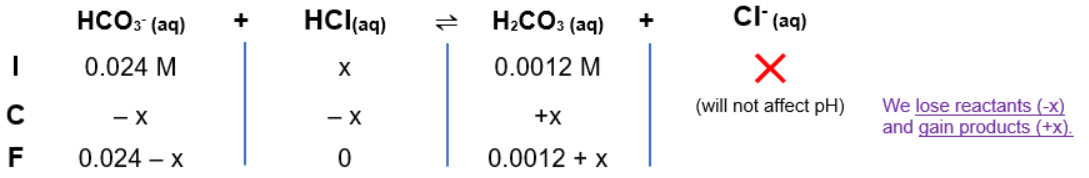# Problem: Blood is buffered by carbonic acid and the bicarbonate ion. Normal blood plasma is 0.024 M in HCO3– and 0.0012 M H2CO3 (pKa1 for H2 CO3 at body temperature is 6.1). If the volume of blood in a normal adult is 5.0 L, what mass of HCl could be neutralized by the buffering system in blood before the pH fell below 7.0 (which would result in death)?

###### FREE Expert Solution

Henderson-Hasselbalch Equation

pH = 7.40 (initial buffer pH)

HCl (strong acid) will react with HCO3- (base).

• base gains H+

Reaction:

HCO3-(aq) + HCl(aq) →H2CO3(aq) + Cl-(aq)

If x = concentration of HCl

ICF CHART:95% (139 ratings)###### Problem Details

Blood is buffered by carbonic acid and the bicarbonate ion. Normal blood plasma is 0.024 M in HCO3 and 0.0012 M H2CO3 (pKa1 for H2 CO3 at body temperature is 6.1). If the volume of blood in a normal adult is 5.0 L, what mass of HCl could be neutralized by the buffering system in blood before the pH fell below 7.0 (which would result in death)?